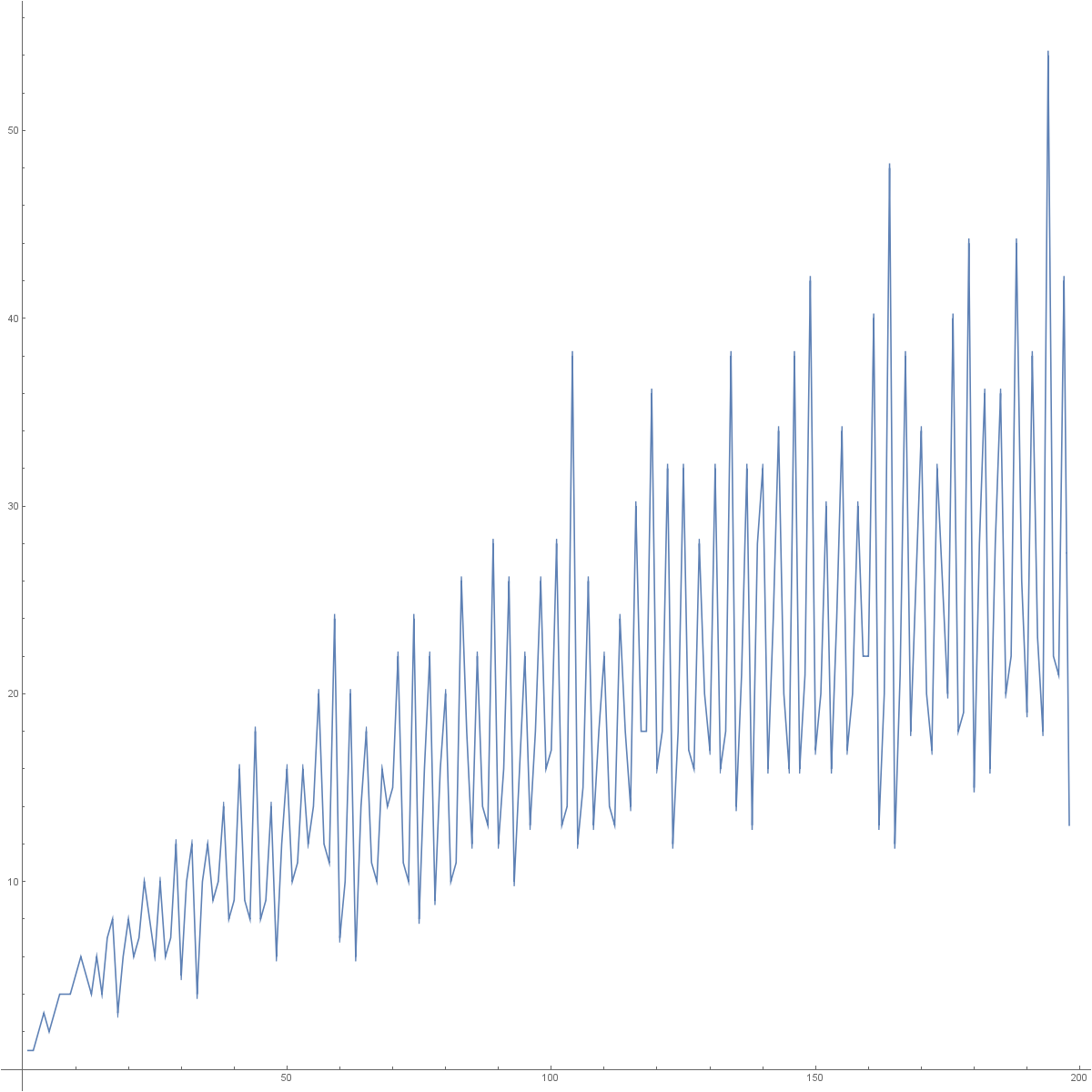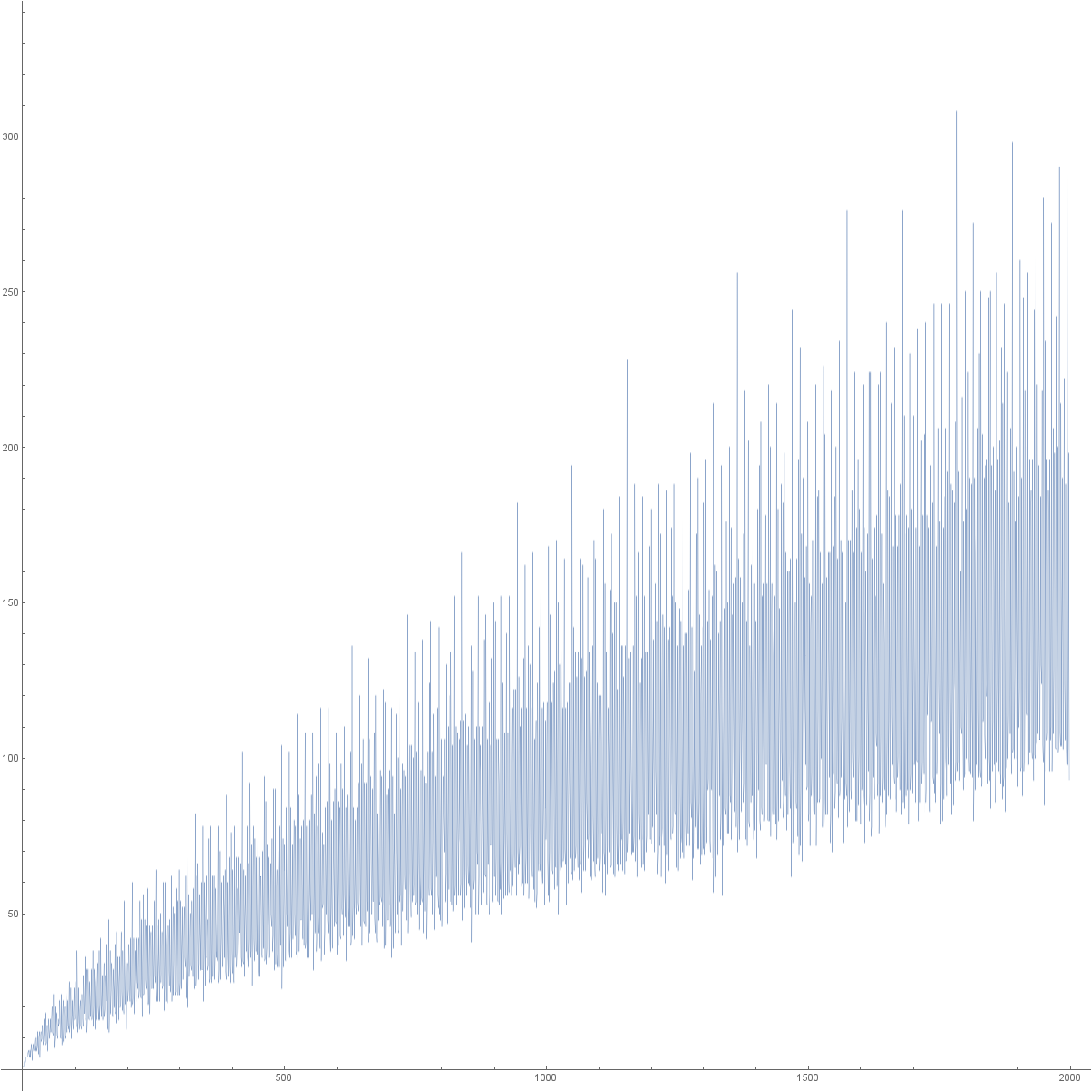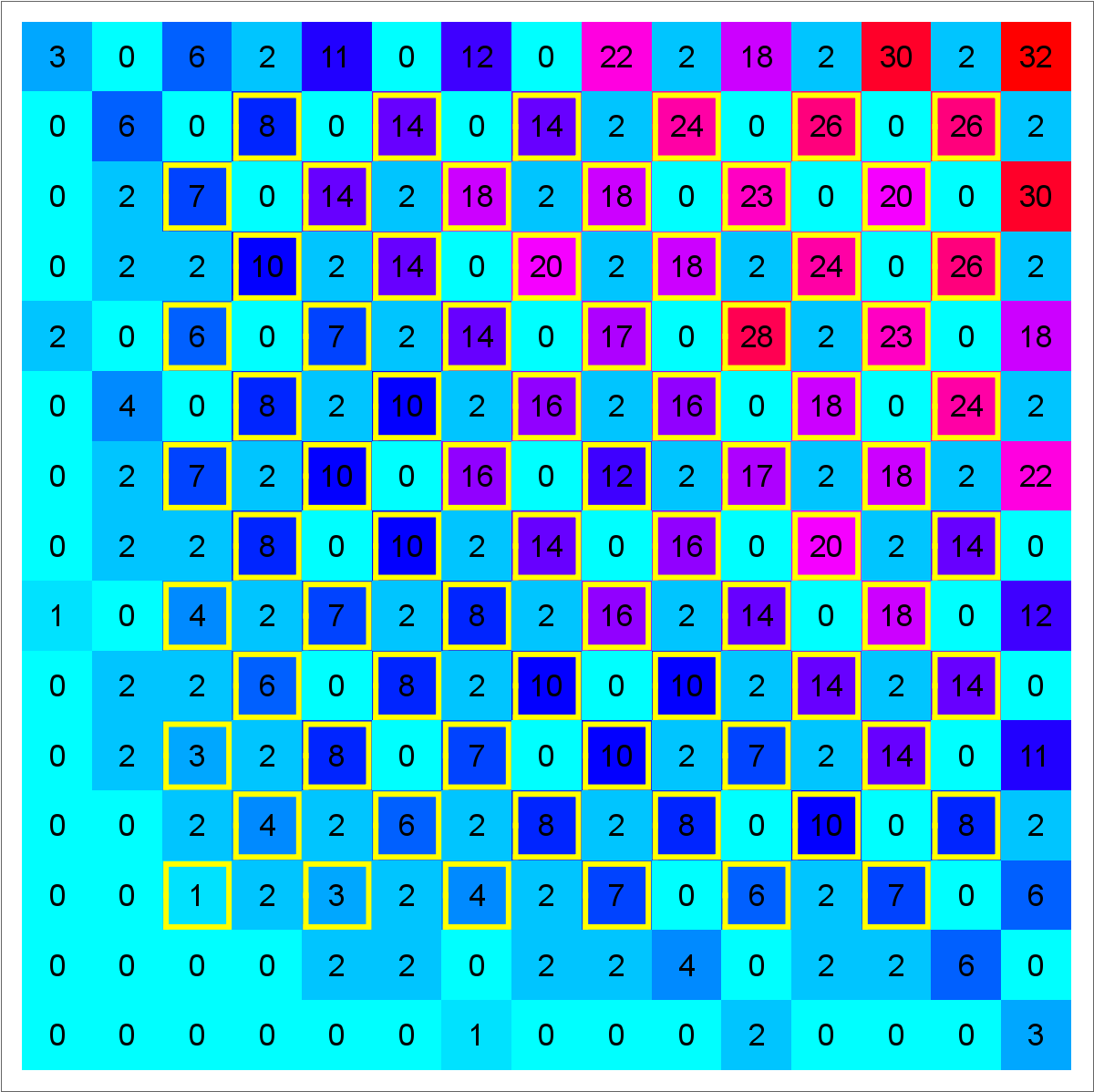# Goldbach

In Unit 18, at the end, you look at the Taylor series f(x) = ∑p xp = x2 + x3 + x5 + .... This function encodes all the primes. Now, the Taylor series of f(x)2 tells in how many ways a number can be written as a sum of two primes. So, in order to see these numbers, we form the function f(x) by taking say up to say n=40: f(x) = x2 + x3 + x5 + x7 + x11 + x13 + x17 + x19 + x23 + x29 + x31 + x37 then form g(x) = f(x)2 to get g(x) = x4 + 2 x5 + x6 + 2 x7 + 2 x8 + 2 x9 + 3 x10 + 2 x12 + 2 x13 + 3 x14 + 2 x15 + 4 x16 + 4 x18 + 2 x19 + 4 x20 + ... We see for example that 10 can be written in three ways as a sum of two primes: 3+7, 7+3, 5+5. This number can be obtained by taking the 10th derivative of g and divide by 10! The Goldbach conjecture asks that all even derivatives of g are positive. It is still unsolved. If you want to experiment, here is the Mathematica code which gives the numbers. We take n=400 which allows us to see all the terms up to n/2 = 200. If we plot this, we see what one calls the Goldbach comet.
 ```n=400; f=Sum[If[PrimeQ[a],x^a, 0], {a,n}]; g=Expand[f*f]; G=CoefficientList[g,x]; s=Table[G[[2 k-1]],{k,3,n/2}]; ListPlot[Flatten[s],AspectRatio->1,Joined->True] ```
 The pictures show the comet for n/2=200 and n/2=400.Are there a multivariable versions? Yes, one can look at Gaussian primes which are Gaussian integers a+i b. The analog claim is that if we look at f(x,y) = ∑p=a+ib,a>0,b>0 xa yb, then all the Taylor entries an,m of g=f*f are positive if n+m is even, bigger than 2. This is the Gaussian Goldbach conjecture. See this project and Paper. We know that the conjecture is hard to prove if it is true as it implies as a special case that there are infinitely many rational primes of the form n2 + 1, which is the Landau conjecture, which is widely considered to be a difficult problem. In any case, the problem is also a problem in calculus, because we are interested in the Taylor expansion of a concrete function g(x,y) of two variables. Like in this story told at the occasion of the 300th birthday of Euler, one can try to find some positive constants c(n,m) such that f(x,y) = ∑p=a+ib,a>0,b>0 c(a,b) xa yb is expressible using known functions like exp, sin, cos, etc, then just compute the derivatives of f(x,y)^2 and establish so the conjecture. Unfortunately, no such choice of coefficients have been found (and in the classical Goldbach case, one has tried since more than 100 years).
 ```n=20; f=Sum[If[PrimeQ[a+I b,GaussianIntegers->True],x^a y^b,0],{a,n},{b,n}]; g=Expand[f*f]; G = CoefficientList[Expand[f*f],{x,y}]; s=Table[G[[i,j]],{i,3,n/2},{j,3,n/2}] ```
Here is the start of the function f(x,y) = x*y + x2*y + x4*y + x*y2 + x3*y2 + x5*y2 + x2*y3 + x*y4 + x5*y4 + x2*y5 + x4*y5 ... The function g(x,y) = f(x,y)2 now starts as g(x,y) = x^2*y^2 + 2*x^3*y^2 + x^4*y^2 + 2*x^5*y^2 + 2*x^6*y^2 + x^8*y^2 + 2*x^2*y^3 + 2*x^3*y^3 + ... We see the coefficients in the following Goldbach matrix.